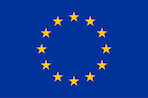# Toposes as bridges between geometric forms and linguistic presentations - A Short Course

I warmly invite you to attend the following short course:

Speaker: Laurent Lafforgue (Huawei Fundamental Research Center, Boulogne-Billancourt, France).

Title: Toposes as bridges between geometric forms and linguistic presentations.

Abstract: The central theme of these lectures is the duality between geometry and languages that Grothendieck toposes realise in mathematical form. The existence of such a duality is enough to make toposes and sites objects of major importance for mathematics and beyond.

Slides of Lectures: Slides

Lecture I: Grothendieck topologies, sheaves, toposes and points.

Date: 18 February 2022 (Friday) at 14:00-15:00 (London time).

Abstract: The purpose of this first lecture will be to introduce the notion of Grothendieck topology on a category, the associated notions of sheaves and toposes, and the derived notion of point of a topos. A special attention will be given to the problem of generation of Grothendieck topologies which, as will become clear in the third lecture, is of great significance and magnitude. Comparing the representations of objects of an arbitrary topos in terms of a presenting site and their evaluations at points provides some extremely general form of non-linear Fourier decompositions and Fourier transforms.

Video: Lecture I

Lecture II: Linguistic descriptions of points and first-order geometric theories.

Date: 25 February 2022 (Friday) at 14:00-15:00 (London time).

Abstract: It will be shown how any presentation of a Grothendieck topos by a site allows to give a linguistic description of set-based points and generalised points of a topos. These descriptions make up bridges from geometry to languages, i.e. to words and grammar rules. They also provide a good way to introduce the general notions of first-order languages and first-order geometric theories.

Video: Lecture II

Lecture III: Classifying toposes, toposes as bridges and the equivalence between first-order provability and generation of Grothendieck topologies.

Date: 4 March 2022 (Friday) at 14:00-15:00 (London time).

Abstract: It will be shown that any geometric first-order theory defines a "functor of models" which associates to any topos the category of the models of this theory with coefficients in this topos, and that this functor of models is always representable by a so-called "classifying topos": it is characterized by the property that models of the theory identify with points of this associated classifying topos. The theory of classifying toposes, which was developped by W. Lawvere and the school of categorical logic in the 1970's, building on some seminal ideas of Grothendieck, was given new impetus with the technique of "toposes as bridges" introduced by O. Caramello in her 2009 PhD thesis. This technique consists in exploiting the fact that any topos can be represented by a double infinite diversity of presenting sites and of geometric theories, in order to develop a general theory of relations between mathematical theories. A particular example of that is the interpretation in terms of quotient theories of the invariant of toposes consisting in their ordered sets of subtoposes. It provides an equivalence between the general problem of provability in the context of first-order geometric theories and the problem of generation of Grothendieck topologies on small categories.

Video: Lecture III